CBSE Class 12 Sample Paper for 2019 Boards

Class 12
Solutions of Sample Papers and Past Year Papers - for Class 12 Boards

Question 29

The members of a consulting firm rent cars from three rental agencies: 50% from agency X, 30% from agency Y and 20% from agency Z. From past experience it is known that 9% of the cars from agency X need a service and tuning before renting, 12% of the cars from agency Y need a service and tuning before renting and 10% of the cars from agency Z need a service and tuning before renting. If the rental car delivered to the firm needs service and tuning, find the probability that agency Z is not to be blamed.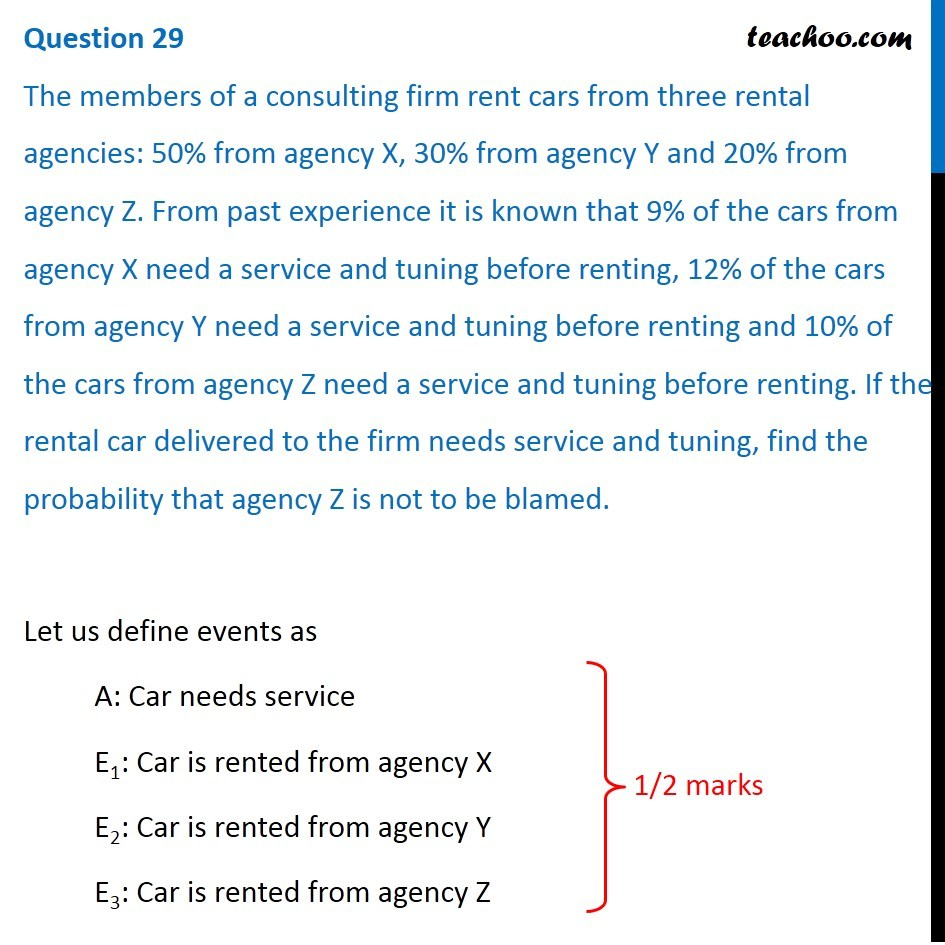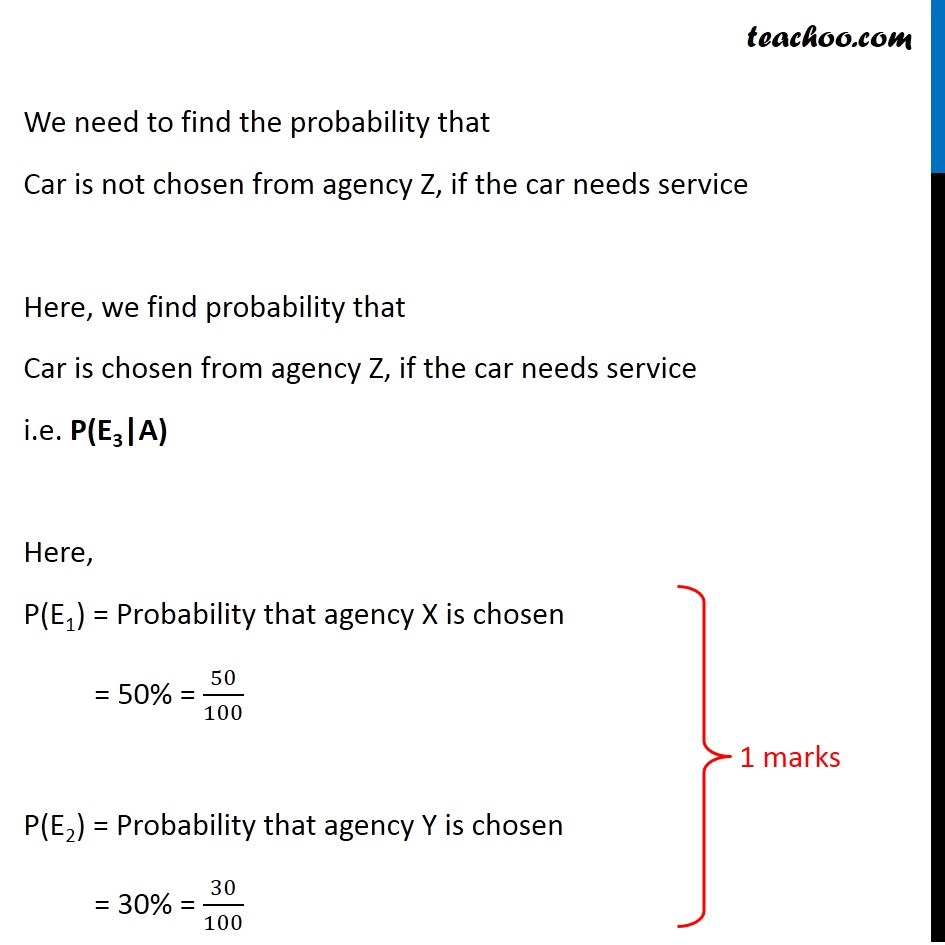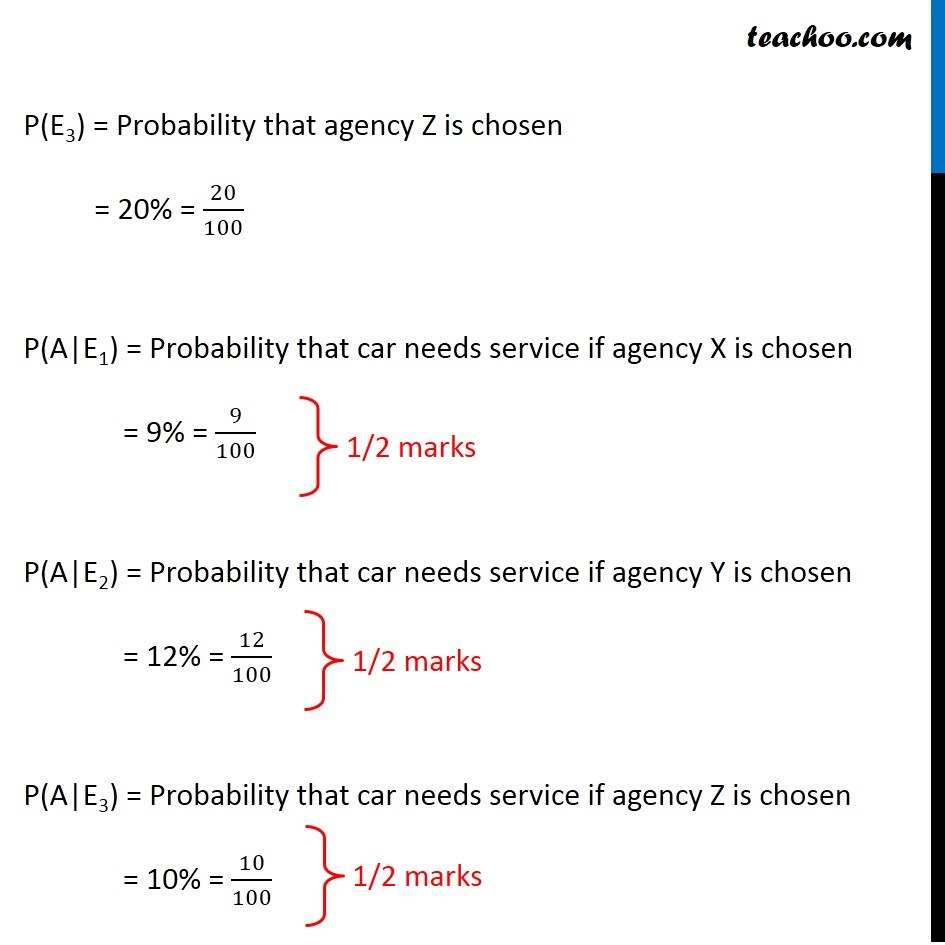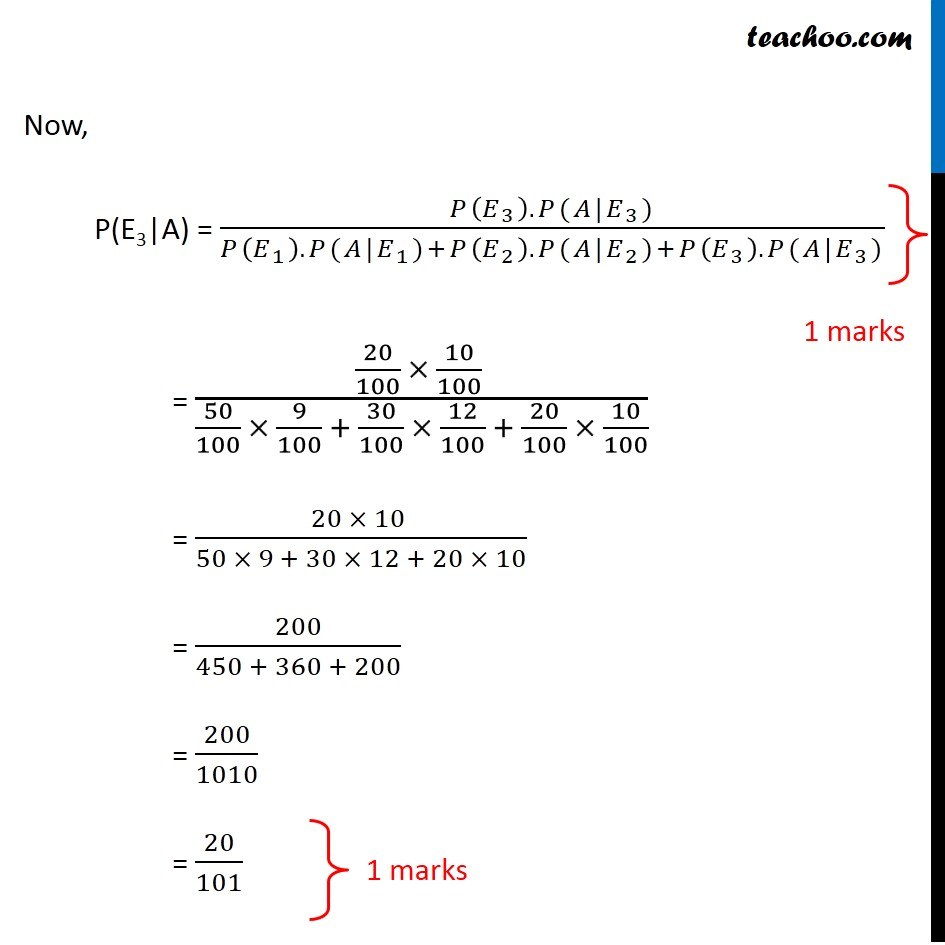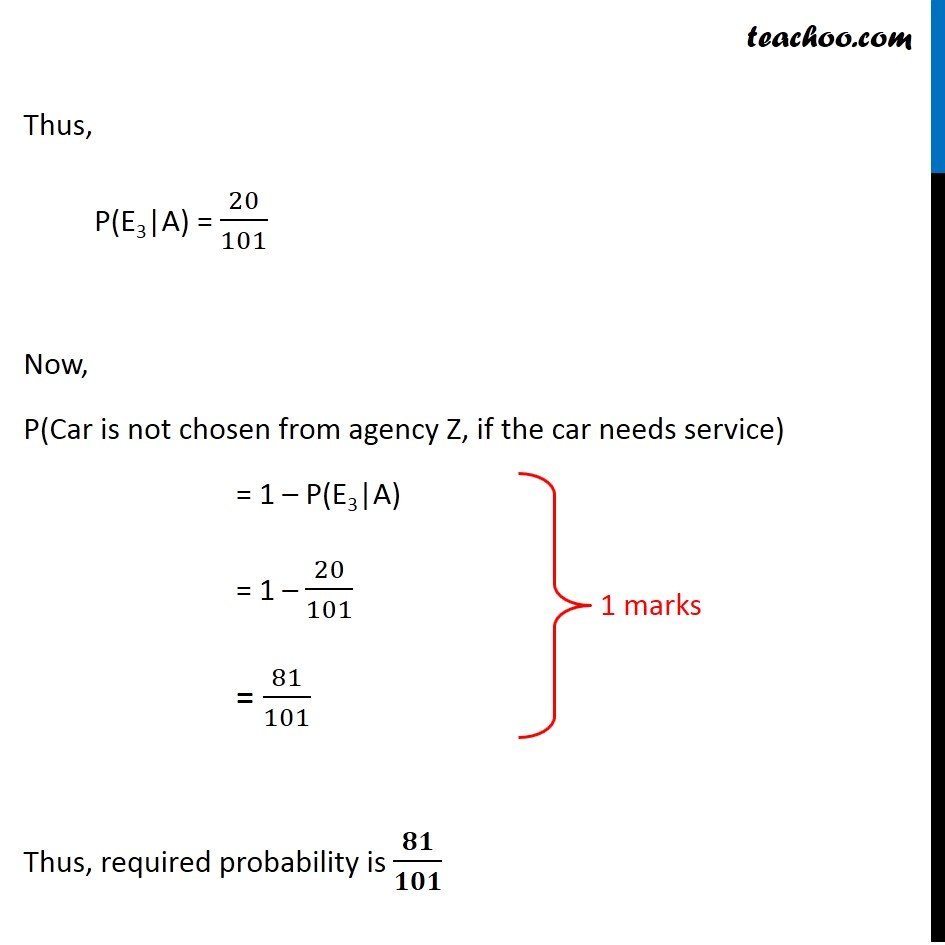Learn in your speed, with individual attention - Teachoo Maths 1-on-1 Class

### Transcript

Question 29 The members of a consulting firm rent cars from three rental agencies: 50% from agency X, 30% from agency Y and 20% from agency Z. From past experience it is known that 9% of the cars from agency X need a service and tuning before renting, 12% of the cars from agency Y need a service and tuning before renting and 10% of the cars from agency Z need a service and tuning before renting. If the rental car delivered to the firm needs service and tuning, find the probability that agency Z is not to be blamed. Let us define events as A: Car needs service E1: Car is rented from agency X E2: Car is rented from agency Y E3: Car is rented from agency Z We need to find the probability that Car is not chosen from agency Z, if the car needs service Here, we find probability that Car is chosen from agency Z, if the car needs service i.e. P(E3|A) Here, P(E1) = Probability that agency X is chosen = 50% = 50/100 P(E2) = Probability that agency Y is chosen = 30% = 30/100 P(E3) = Probability that agency Z is chosen = 20% = 20/100 P(A|E1) = Probability that car needs service if agency X is chosen = 9% = 9/100 P(A|E2) = Probability that car needs service if agency Y is chosen = 12% = 12/100 P(A|E3) = Probability that car needs service if agency Z is chosen = 10% = 10/100 Now, P(E3|A) = (𝑃(𝐸_3 ).𝑃(𝐴|𝐸_3))/(𝑃(𝐸_1 ).𝑃(𝐴|𝐸_1)+𝑃(𝐸_2 ).𝑃(𝐴|𝐸_2)+𝑃(𝐸_3 ).𝑃(𝐴|𝐸_3)) = (20/100 × 10/100 )/(50/100 × 9/100 + 30/100 × 12/100 + 20/100 × 10/100) = (20 × 10 )/(50 × 9 + 30 × 12 + 20 × 10) = 200/(450 + 360 + 200) = 200/1010 = 20/101 Thus, P(E3|A) = 20/101 Now, P(Car is not chosen from agency Z, if the car needs service) = 1 – P(E3|A) = 1 – 20/101 = 81/101 Thus, required probability is 𝟖𝟏/𝟏𝟎𝟏